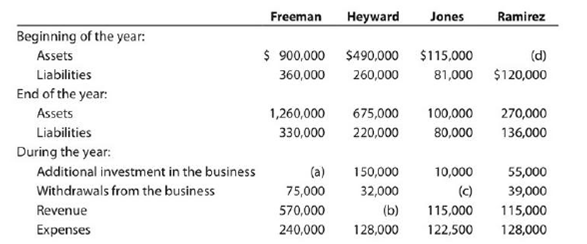Chapter 1, Problem 1.20EX### Accounting (Text Only)

26th Edition
Carl Warren + 2 others
ISBN: 9781285743615

#### Solutions

Chapter
Section### Accounting (Text Only)

26th Edition
Carl Warren + 2 others
ISBN: 9781285743615
Textbook Problem
3637 views

# Missing amounts from balance sheet and income statement dataOne item is omitted in each of the following summaries of balance sheet and income statement data for the following four different proprietorships:Determine the missing amounts, identifying them by letter. (Hint: First, determine the amount of increase or decrease in owner's equity during the year.)

To determine

Income statement: The financial statement which reports revenues and expenses from business operations and the result of those operations as net income or net loss for a particular time period is referred to as income statement.

Balance sheet: This financial statement reports a company’s resources (assets) and claims of creditors (liabilities) and stockholders (stockholders’ equity) over those resources. The resources of the company are assets which include money contributed by stockholders and creditors. Hence, the main elements of the balance sheet are assets, liabilities, and stockholders’ equity.

To Determine:  The missing amount in the given statements.

Explanation

a)

The additional investment of Company F is calculated below.

 Stockholders’ equity at end of year (1) $930,000 Stockholders’ equity at beginning of year (2)$540,000 Increase in stockholders’ equity $390,000 Deduct increase due to net income (3)$330,000 Increase due to additional investments less withdrawals $60,000 Add withdrawals$75,000 Additional common stock issued $135,000 Table (1) Hence, the additional investment of Company F is$135,000.

Working Note:

Calculate the Stockholders’ equity at end of year:

Stockholders’ equityat end of year}= Assets at end of yearLiabilities at end of year= $1,260,000$330,000=$930,000 (1) Calculate the Stockholders’ equity at beginning of year: Stockholders’ equityat beginning of year}(Assets at beginningof year)(Liabilities at beginningof year)=$900,000 $360,000=$540,000

(2)

Calculate the Net Income during the year:

Net Income= Revenue  Expenses= $570,000$240,000=$330,000 (3) b) The revenue of Company H is calculated below.  Stockholders’ equity at end of year (4)$455,000 Stockholders’ equity at beginning of year  (5) 230,000 Increase in stockholders’ equity $225,000 Add withdrawals 32,000 Increase due to additional investment and net income$257,000 Deduct Additional investment 150,000 Increase due to Net income $107,000 Add expenses 128,000 Revenue$235,000

Table (2)

Hence, the revenue of Company H is $235,000. Working Note: Calculate the Stockholders’ equity at end of year: Stockholders’ equityat end of year}= Assets at end of yearLiabilities at end of year=$675,000 $220,000=$455,000

(4)

Calculate the Stockholders’ equity at beginning of year:

Stockholders’ equityat beginning of year}(Assets at beginningof year)(Liabilities at beginningof year)= $490,000$260,000=\$230,000

(5)

c)

The withdrawals from Company J are calculated below

### Still sussing out bartleby?

Check out a sample textbook solution.

See a sample solution

#### The Solution to Your Study Problems

Bartleby provides explanations to thousands of textbook problems written by our experts, many with advanced degrees!

Get Started

#### Find more solutions based on key concepts